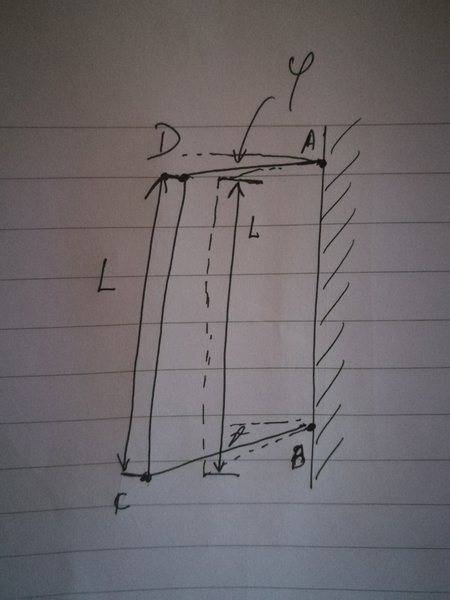# Missing one equation to solve the linear system

• skrat

## Homework Statement

So imagine 4 rigid rods connected together to form a dashed quadrilateral as shown in the picture.Now AB is fixed, can not be changed in anyway, while all other sides (AD, BC and CD) are connected but can move freely. The initial conditions (dashed quadrilateral) are given.
Now we rescale the dashed BC and AD sides to given values. Note that the length of CD remains constant!

Find coordinates of points C and D of newely generated quadrilateral in such way that the slope of AD and BC is minimally changed.

## The Attempt at a Solution

Ok so the way I see the problem I have 4 values to determine
$$(x_C, y_C)\text{ and } (x_D, y_D).$$
First 3 (out of 4) equations are
$$||\vec{BC}|| = \sqrt{(x_C - x_B)^2 + (y_C - y_B)^2}$$
$$||\vec{AD}|| = \sqrt{(x_D - x_A)^2 + (y_D - y_A)^2}$$
$$||\vec{DC}|| = \sqrt{(x_C - x_D)^2 + (y_C - y_D)^2}$$

The forth one is the one I am struggling with. I know that the slope equals ##\tan \varphi = \frac{\Delta x}{\Delta y}## but I'm not really sure what to do with that. I'm quite sure I need to find the relation between ##\vartheta## and ##\varphi## first.

EDIT: This probably doesn't lead to a linear system of equations. I assume Lagrangian multiplier will be needed or some other method for finding multivariate extremes. I will kindly ask the moderators to change the topic title.

#### Attachments

Last edited by a moderator:

Now we rescale the dashed BC and AD sides to given values.
It's not clear what you mean by that.

in such way that the slope of AD and BC is minimally changed.
By the "slope of AD" , do you mean ##\tan (\theta)##? It isn't clear why ##\theta## would need to change at all when you "rescale" AD and BC.

It's not clear what you mean by that.
Lengthen. From ##||\vec {BC}||## to ##||\vec{BC}||'##.

By the "slope of AD" , do you mean ##\tan (\theta)##? It isn't clear why ##\theta## would need to change at all when you "rescale" AD and BC.
Sorry, it is not clear. You are correct, ##\tan \vartheta## is not allowed to change when lengthening the sides. It's totally unnecessary to specifically point that out.

Find coordinates of points C and D of newely generated quadrilateral in such way that the slope of AD and BC is minimally changed.
So by "minimally changed" you meant "unchanged"?

So by "minimally changed" you meant "unchanged"?
That would be amazing, but I don't see how that would be possible except in special cases.

Note that the length of CD is constant, while sides AD an BC are (except in special cases) NOT paralel. So from my understanding, the angles are unchanged if and only if AD and BC are parallel and if both of the sides are lengthen by the same amount.

When you said ##\tan\theta## is not allowed to change in post #3, that's the same as saying the slope of BC can't change, but in the original post, you say the slope of BC can change.

If both ##\theta## and ##\phi## can change, what precisely do you mean by minimally changed?

When you talk about rescaling BC and AD, do you mean by the same factor, or could they change length independent of each other?

•Delta2
When you said ##\tan\theta## is not allowed to change in post #3, that's the same as saying the slope of BC can't change, but in the original post, you say the slope of BC can change.
Yeah that's a result of my bad english and poor problem statement.
So
2. Then we rescale the BC and AD - almost never for the same factor, except in special cases. The BC side is rescaled along vector ##\hat{BC}## and AD along ##\hat{AD}##. So "the slope of BC and AD can't change" applies to this step ONLY. I think this is more clear now.
3. Since the AD and BC sides were lengthened (and since they are in general not parallel to each other), the length of CD is now either to long or to short - the OP says the length of CD has to remain constant - I have to rotate points C and D to change the distance between them. And I want to rotate them in such a way that the slope of BC and AD is minimally changed.

If both ##\theta## and ##\phi## can change, what precisely do you mean by minimally changed?
That's the tricky part, left up to me to decide. Since ##\theta## and ##\phi## are not independent it might be sufficient to minimize their sum, if not, maybe sum of the squares of the slope, ...
If possible I would like to find an analytical solution to the problem, so the challenge is to find a condition that:
- minimally changes the shape of the quadrilateral (it was my interpretation that this means minimal change of the BC and AD slopes, maybe you have a better idea)
- possibly brings me to an analytical solution (but don't bother with that too much)

•Delta2
Anybody?

minimally changes the shape of the quadrilateral (it was my interpretation that this means minimal change of the BC and AD slopes, maybe you have a better idea)
even if you fix on minimal change to slope, that is still open to imterpretation. How about rotation of each through the smallest angle, ψ (same angle for each but opposite directions)? Of course, there might be no such solution. Indeed, if one rod is extended much more than the other there may be no solution at all.